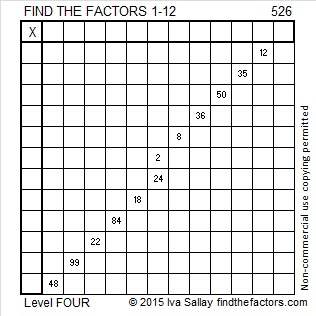# 526 is a Centered Pentagonal Number

I am excited that Kerrydunton included the Find the Factors puzzles on a list of great maths starters.

1 + 5 + 10 + 15 + 20 + 25 + 30 + 35 + 40 + 45 + 50 + 55 + 60 + 65 + 70 = 526. Thus, 526 is the 15th centered pentagonal number.

The above equation is the same as saying that 526 is one more than five times the 14th triangular number.The last digit of a centered pentagonal number is always 1 or 6. Triangular numbers greater than 3 are always composite numbers, but centered pentagonal numbers ending in 1 might be prime numbers. For example 31, 181, 331, 601, 1051, 1381, and 3331 are centered pentagonal numbers and prime numbers.Print the puzzles or type the solution on this excel file: 12 Factors 2015-06-15

—————————————————————————————————

• 526 is a composite number.
• Prime factorization: 526 = 2 x 263
• The exponents in the prime factorization are 1 and 1. Adding one to each and multiplying we get (1 + 1)(1 + 1) = 2 x 2 = 4. Therefore 526 has exactly 4 factors.
• Factors of 526: 1, 2, 263, 526
• Factor pairs: 526 = 1 x 526 or 2 x 263
• 526 has no square factors that allow its square root to be simplified. √526 ≈ 22.93468988—————————————————————————————————